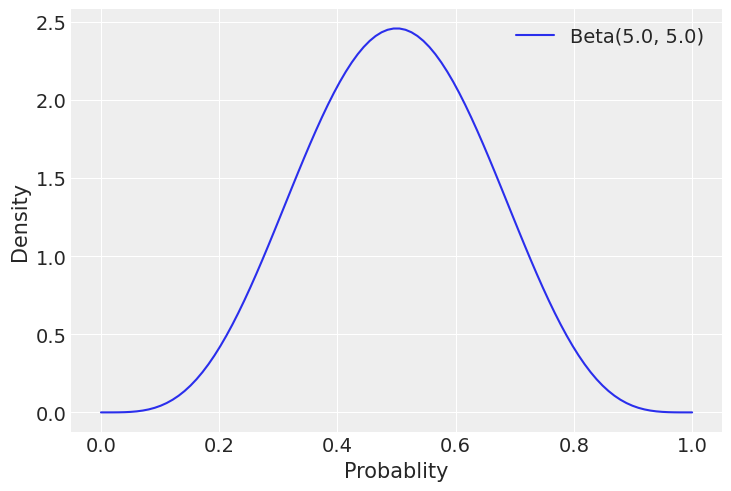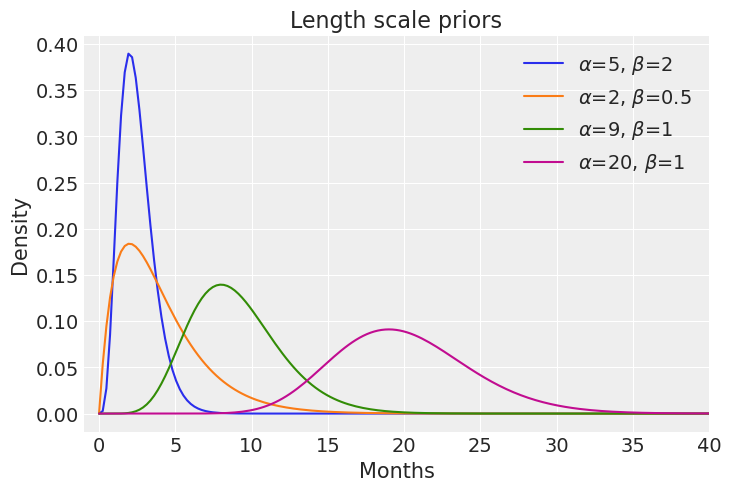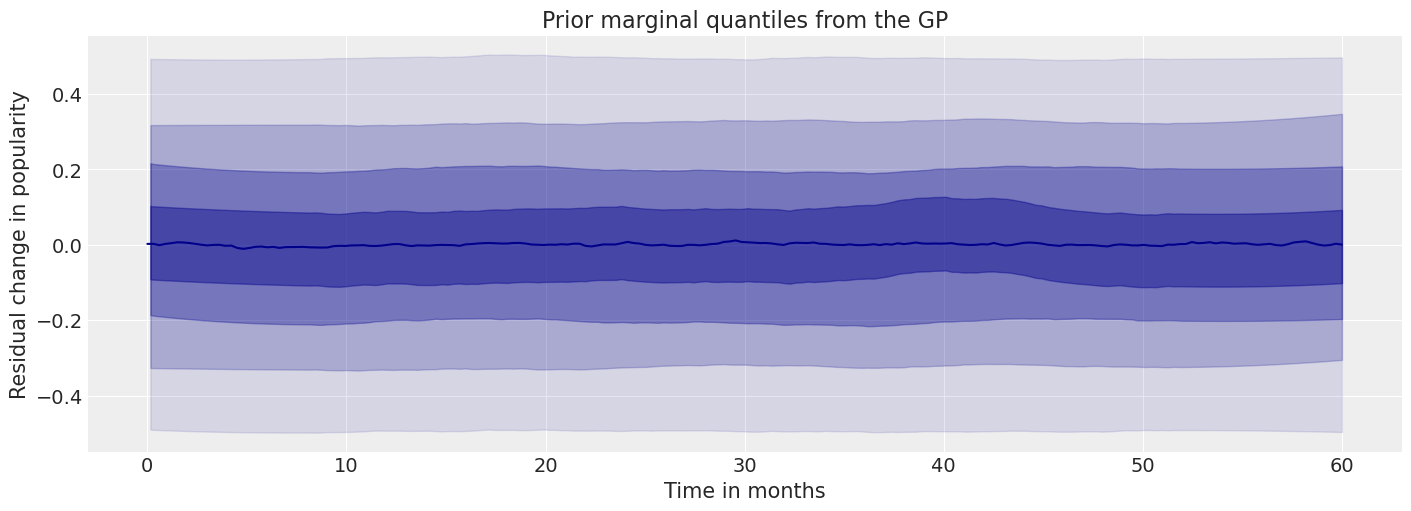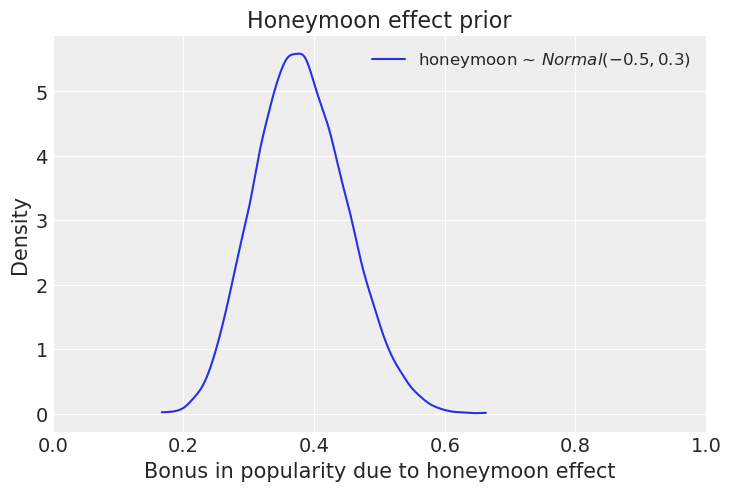Note: This is a blog post detailing how the GP model of popularity is built. To access the interactive dashboard of the model’s predictions, click here.

I like working on time series, because they usually relate to something concrete. I've also long been intrigued by Gaussian Processes -- they have a mathematical beauty and versatility that I've always found intriguing, if only because you can parametrize the model in ways where you can interpret it.

But... they are hard to fit -- the number of gradient computation scales with the cube of the number of data points. And in the Bayesian framework, we're trying to estimate the whole distribution of outcomes, not only one single point, which adds to the challenge.

One thing I learned so far in my open-source programming journey is not to be afraid of what you're afraid of -- to what you'll legitimately answer: "wait, what??". Let me rephrase: if a method scares you, the best way to understand it is to work on an example where you need it. This will dissipate (part of) the magic behind it and help you cross a threshold in your understanding.

So that's what I did with Gaussian Processes! That all came from a simple question: how does the popularity of French presidents evolve within term and across terms? I often hear people frenetically commenting the latest popularity poll (frequently the same people who later will complain that "polls are always wrong", but that's another story), and in these cases I'm always worried that we're reacting to noise -- maybe it's just natural that a president experiences a dip in popularity at the middle of his term?

To answer this question, I compiled all the popularity opinion polls of French presidents since the term limits switched to 5 years (in 2002). Let's see what the data look like, before diving into the Gaussian Process model.

## Show me the data!

Here are the packages we'll need:

import arviz as az
import matplotlib.pyplot as plt
import numpy as np
import pandas as pd
import pymc3 as pm
import xarray as xr
from scipy.special import expit as logistic


Now, let's load up the data in a dataframe called d. You'll notice that, in addition to the polling data, the dataframe also contains the quarterly unemployment rate in France (downloaded from the French statistical office). As this variable is usually well correlated with politicians' and parties' popularity, we will use it as a predictor in our model.

As I'll explain below, we're computing the popularity every month, but since unemployment data are released quarterly, we just forward-fill the unemployment values when they are missing -- which is, I insist, an assumption, and as such it should be tested and played with, to check its impact on the model's inferences (there is a Binder and Google Collab link at the top of the page, so feel free to do so!). I could also use more intricate techniques to forecast unemployment, but that would be an overkill for our purpose here.

level_0 president samplesize p_approve p_disapprove party election_flag N_approve N_disapprove N_total unemployment
month
2002-05-31 2002Q2 chirac2 964.250000 0.502500 0.442500 right 1 485 427 912 7.5
2002-06-30 2002Q2 chirac2 970.000000 0.505000 0.425000 right 0 490 412 902 7.5
2002-07-31 2002Q3 chirac2 947.333333 0.533333 0.406667 right 0 505 385 890 7.5
2002-08-31 2002Q3 chirac2 1028.000000 0.520000 0.416667 right 0 535 428 963 7.5
2002-09-30 2002Q3 chirac2 1017.500000 0.525000 0.420000 right 0 534 427 961 7.5
... ... ... ... ... ... ... ... ... ... ... ...
2020-12-31 2020Q4 macron 1156.500000 0.388333 0.590000 center 0 449 682 1131 7.7
2021-01-31 2021Q1 macron 1184.181818 0.380000 0.589091 center 0 450 698 1148 7.7
2021-02-28 2021Q1 macron 1128.625000 0.405000 0.576250 center 0 457 650 1107 7.7
2021-03-31 2021Q1 macron 1100.545455 0.375455 0.582727 center 0 413 641 1054 7.7
2021-04-30 2021Q2 macron 1001.666667 0.350000 0.596667 center 0 351 598 949 7.7

228 rows × 11 columns

If you go check the raw underlying notebook, you'll see that there is a bunch of data cleaning involved to get to that format. The most important is that we perform a simple monthly average to get fixed time intervals, which makes computation easier for the GP -- and it's actually not that far-fetched to consider that we get to "observe" the president's popularity only once a month, thanks to the average of all polls taken in this month.

Ideally though, we wouldn't do that, as 1) it breaks the purely generative aspect of the model (now the model doesn't take as observations the raw polls but their average), and 2) it tricks the model into believing that the dispersion in polls is lower than it actually is.

As a first implementation though, let's make our lives easier and see how that goes -- we can always go back to the model and relax this assumption if needed.

Speaking of making our lives easier, let's write a helper function to convert datetimes to numbers, in reference to a given date. This will be useful to use time as a predictor in our model -- more precisely, as an input to our GP (completely lost? Don't worry, we'll get back to that).

def dates_to_idx(timelist):
"""Convert datetimes to numbers in reference to a given date"""

reference_time = timelist
t = (timelist - reference_time) / np.timedelta64(1, "M")

return np.asarray(t)

time = dates_to_idx(d.index)
time[:10]

array([0.        , 0.98564652, 2.00414793, 3.02264934, 4.00829586,
5.02679726, 6.01244379, 7.03094519, 8.0494466 , 8.96938335])

Let's also define a function to standardize data (mean 0 and standard deviation 1), which we'll use for our only continuous predictor, the unemployment rate. Indeed that will make it easier to set our priors for the coefficient associated to unemployment, and our sampler will have a better time sampling -- so, you know two 🐦 with one 💎 !

def standardize(series):
"""Standardize a pandas series"""
return (series - series.mean()) / series.std()


## Build me a model

Now is time to define the model, which should make things clearer. The polls are, quite simply, realizations of a Binomial distribution: for each poll, a number $n$ of people are surveyed, and $y$ of them say they approve of the president's job. Note that we ignore those who report no opinion, because their numbers are usually negligeable, so $n - y$ represents the number of people who disapprove of the president's job. Statistically speaking, we have $y \sim Binomial(n, p)$, where $p$ equals the proportion of people supporting the president.

$p$ is really what we're after here: given the observed polls, what is our inference of the true, latent support of the president in the population? But I can feel that something is bothering you, isn't it (yeah, I can read minds)? Right now you're thinking: "but aren't polls noisy observations of reality"? To what I'll answer: "boy, you're really good at this!". Polls are indeed noisy estimates and a variety of factors account for these polling errors.

So, we need to take that into account, and a quick way to do that is to use the Beta-Binomial distribution, which handles overdispersed data -- i.e observations that are more variable than a classic Binomial distribution would expect and can accomodate. If you want more details about these models (poetically named "continuous mixture models"), I'd refer you to chapter 12 of Richard McElreath's excellent Statistical Rethinking.

In the Beta-Binomial distribution, the Binomial probabilities are no longer fixed, but are rather random variables drawn from a common Beta distribution. So, in addition to the number of trials, $n$, Beta-Binomial distributions are parametrized by two strictly positive reals, $\alpha$ and $\beta$, which control the Beta distribution.

The thing is that $\alpha$ and $\beta$ usually don't have a real meaning, so it's often easier to parametrize the Beta distribution with two other parameters, $p$ and $\theta$, which roughly correspond to the mean and precision respectively: for a given $p$, a higher $\theta$ means that we are more skeptical of very weak or very strong probabilities -- those near 0 or 1.

We don't need a prior on $p$, as it will be a deterministic function of our regression. But we do need one for $\theta$. A value of 2 translates in a uniform prior over probabilities, which is not what we want -- we know presidential approval never goes below 10% and above 90% (at least in France). To get that, we can set $\theta = 10$. You can play around with the code below to get a sense of how the Beta family behaves:

x_plot = np.linspace(0, 1, 100)
pbar = 0.5
theta = 10.0

plt.plot(
x_plot,
np.exp(pm.Beta.dist(pbar * theta, (1 - pbar) * theta).logp(x_plot).eval()),
label=f"Beta({pbar * theta}, {(1 - pbar) * theta})",
)
plt.xlabel("Probablity")
plt.ylabel("Density")
plt.legend();Looks good right? And you can see that the mathematical link between $(p, \theta)$ and $(\alpha, \beta)$ is quite simple:

$$\alpha = p \times \theta$$ $$\beta = (1 - p) \times \theta$$

So, we want to assume that the precision is at least 10. To that end, we can use a trick and define $\theta = \tilde{\theta} + 10$, where $\tilde{\theta} \sim Exponential(1)$, which works because exponential distributions have a minimum of zero.

Let's turn our attention to $p$ now, the parameter we really care about. We model it through the addition of a linear component ($baseline + \beta_{honeymoon} \times election\_ flag + \beta_{unemp} \times unemp\_ data$ and a non-parametric component (the GP, $f\_time$). The GP basically allows us to tell the model that time and popularity covary, but we don't know the exact functional form of this romantic relationship, so we'd like the model to figure it out for us -- yep, GPs are pretty cool; I bet they were popular in college! To make sure $p$ stays between 0 and 1 (it's a probability, remember?), we use the logistic link function.

We now have all the parts to build our model! We just need to define the priors for all our unknown parameters. That what we'll do in the next section, but first, let's assemble all the building blocks, to contemplate our beautiful inference machine (yeah, I took a couple poetry classes in highschool):

$$y \sim BetaBinomial(\alpha=p \times \theta, \: \beta=(1 - p) \times \theta, \: n)$$

$$theta \sim Exponential(1) + 10$$

$$p = logistic(baseline + f\_ time + \beta_{honeymoon} \times election\_ flag + \beta_{unemp} \times log(unemp\_ data))$$

$$baseline \sim Normal(-0.7, 0.5)$$

$$\beta_{honeymoon} \sim Normal(-0.5, 0.3)$$

$$\beta_{unemp} \sim Normal(0, 0.2)$$

$$f\_ time \sim GP(0, \Sigma)$$

$$\Sigma = amplitude^2 \times Matern52(length\_ scale)$$

$$amplitude \sim HalfNormal(1)$$

$$length\_ scale \sim Gamma(\alpha=5, \beta=2)$$

As I know you're very attentive, you have noticed that we use the logarithm of unemployment, not the raw unemployment rate. It's because we think that what matters for citizens when they think about unemployment is its order of magnitude, not its absolute values.

Other than that, the model should look pretty familiar to you now -- well, except for the priors on the GP and the coefficients. So, let's turn to that right now!

## Time to choose... your GP prior

Priors are very important to fit GPs properly, so let's spend some time thinking about our priors for a more refined model of the popularity of the president. Note that this is a tutorial about how to code up a GP in PyMC3, not a tutorial about the theory of GPs, so we assume familiarity with the concepts. If you need a refresher about the theory, take a look at PyMC3's Mauna Loa notebook, Michael Betancourt's excellent case study and chapter 21 of Bayesian Data Analysis.

### Kernel relations

We will use a fairly common Matern 5/2 kernel. It has the advantage of being less smooth than the classic exponentiated quadratic kernel, which is useful here as the GP must be able to jump from one regime to another very quickly when a new president is sworn in (you'll see that the swing in popularity is usually very wide).

We could probably do something better here by signaling these change points to our GP (after all, we know when a new president comes in, so we should tell it to our model). That way, we could use different kernels for each president. Or we could look into non-stationary kernels: the Matern kernel is stationary, meaning that the estimated covariance between data points doesn't vary depending on the period we're in -- maybe French people's assessment of their presidents varied less (i.e was more autocorrelated) in the 2000s, when there were no 24/7 TV news networks?

In short, it's easy to imagine that the way popularity varies with time (which is what our GP tries to capture) itself varies according to the president's personality and the times he lived in. The improvements above would integrate this assumption into the model. But, again, this is a first version -- let's see if, like the 2019 Nobel Chemistry prize, it's good enough.

### Amplitude -- Don't go so high!

The Matern 5/2 kernel is parametrized with an amplitude and a length scale. The amplitude controls, quite surprisingly, the amplitude of the GP realized values: the bigger the amplitude, the larger the variations in the GP values. You can think of it as the $y$ axis in the plots you will see below -- the range of values that $f(x)$ can take on, $f$ being drawn from the GP.

Proportions in polls vary from 0 to 1, and the GP models only part of the variation in polls. We will also standardize our predictor (unemployment data), which means it will have a standard deviation of 1. So, in this context, $Halfnormal(1)$ should be a weakly regularizing prior.

### Length scale -- Don't go so far!

I know you're quite impatient, so I already hear you asking: "what about the length scale now?". Well, ok! But do you even know what that controls? The length scale can be interpreted as the degree of correlation between the GP values: the higher it is, the smoother the functions drawn from the GP, because their realized values will be highly correlated. In a way, you can think of the length scale as the maximum distance on the $x$ axis at which two points in time still share information.

In our case, we can imagine that polls taken 3 months ago still have a bit of influence on today's results, but it's probably not the case for polls from more than 6 months ago. So we need a prior that's both avoiding 0 (by definition, it never makes sense for the length scale to be exactly 0) and constraining values above about 6 months. The Gamma family of distributions is usually a helpful choice here, as it is very versatile and has support over the positive real line. Here are a few examples, to give you an idea:

x = np.linspace(0, 120, 500)
priors = [
(r"$\alpha$=5, $\beta$=2", pm.Gamma.dist(alpha=5, beta=2)),
(r"$\alpha$=2, $\beta$=0.5", pm.Gamma.dist(alpha=2, beta=0.5)),
(r"$\alpha$=9, $\beta$=1", pm.Gamma.dist(alpha=9, beta=1)),
(r"$\alpha$=20, $\beta$=1", pm.Gamma.dist(alpha=20, beta=1)),
]

fig = plt.figure()

for i, prior in enumerate(priors):
plt.plot(x, np.exp(prior.logp(x).eval()), label=prior)

plt.xlim((-1, 40))
plt.xlabel("Months")
plt.ylabel("Density")
plt.title("Length scale priors")
plt.legend();The blue line, representing $Gamma(5, 2)$ has our favors, because most of the probability mass is between 0 and 6 months, which we deemed reasonable above.

If you're a bit lost, that's quite normal: GPs are rather meta, so it takes some time to develop intuition about them. A nice thing though is that we can relate the GP's parameters to the reality of our use-case, which makes it more interpretable.

In any case, take your time going through this, and understanding will come. This notebook about mean and covariance functions and this case study are good educational ressources to think about priors in the context of GPs.

### Putting the GP together -- You're beautiful!

The best we can do now to make this more concrete is to draw some functions from our GP, prior to seeing any data. That will help us understand our model and determine if our prior choices make sense. This is pretty simple to do with PyMC3:

amplitude_trend = pm.HalfNormal.dist(1.0).random(1)
ls_trend = pm.Gamma.dist(alpha=5, beta=2).random(1)
cov_trend = amplitude_trend ** 2 * pm.gp.cov.Matern52(1, ls_trend)

prior_timepoints = np.linspace(0, 60, 200)[:, None]
K = cov_trend(prior_timepoints).eval()

gp_prior_samples = np.random.multivariate_normal(mean=np.zeros(K.shape), cov=K, size=20_000)

_, (left, mid, right) = plt.subplots(
1, 3, figsize=(14, 5), constrained_layout=True, sharex=True, sharey=True
)
for ax, samples in zip((left, mid, right), (5, 10, 100)):
ax.plot(
prior_timepoints,
gp_prior_samples[:samples].T,
color="darkblue",
alpha=0.3,
)
ax.set_title("Samples from the GP prior")
ax.set_xlabel("Time in months")
ax.set_ylabel("Popularity evolution");Each line is a realization of the GP prior. We indeed see the effect of our priors on both the amplitude and length scale, with most functions fluctuating between -1 and 1 (amplitude) and the auto-correlation being limited to around 6 months (length scale).

As we didn't specify a mean for our GP, PyMC by default centers it at 0, which happens to also be what we want: the GP is here to capture the residual variation of popularity once we've taken into effect the baseline, unemployment and honeymoon effects.

The "spaghetti" plots above are useful to get an idea of individual functions and the correlations between their values, but it's hard to understand exactly where different quantiles end up. To get that information, "ribbon" plots are more interesting. Let's compute and display the median, 40-60%, 30-70%, 20-80% and 10-90% quantile intervals of the GP prior samples we already drew above:

/Users/alex_andorra/opt/anaconda3/envs/elections-models/lib/python3.9/site-packages/arviz/stats/stats.py:456: FutureWarning: hdi currently interprets 2d data as (draw, shape) but this will change in a future release to (chain, draw) for coherence with other functions
warnings.warn(
/Users/alex_andorra/opt/anaconda3/envs/elections-models/lib/python3.9/site-packages/arviz/stats/stats.py:456: FutureWarning: hdi currently interprets 2d data as (draw, shape) but this will change in a future release to (chain, draw) for coherence with other functions
warnings.warn(
/Users/alex_andorra/opt/anaconda3/envs/elections-models/lib/python3.9/site-packages/arviz/stats/stats.py:456: FutureWarning: hdi currently interprets 2d data as (draw, shape) but this will change in a future release to (chain, draw) for coherence with other functions
warnings.warn(
/Users/alex_andorra/opt/anaconda3/envs/elections-models/lib/python3.9/site-packages/arviz/stats/stats.py:456: FutureWarning: hdi currently interprets 2d data as (draw, shape) but this will change in a future release to (chain, draw) for coherence with other functions
warnings.warn(Easier to visualize the different expectations, right? Let's note though that these plots ignore the correlation between time points, so they underestimate the overall variation in the GP samples -- and indeed you can see that the $y$ axis is on a smaller scale than the spaghetti plots'.

## But wait, there is more... priors to choose

Enjoying it so far? Of course you are! Well good news: we still have priors to pick for the intercept, honeymoon and unemployment effects 🍾

Our regression intercept is also the mean function of our GP -- the value it reverts to when data start lacking. There, we have quite a lot of information: 50% popularity is historically high for a French president, so keeping the mean at zero is sub-optimal -- our parameter lives on the logit scale, so a prior centered at 0 means a prior centered at $logistic(0) = 0.5$ on the outcome space.

We can do better: based on our domain knowledge, we expect most presidents to have a baseline popularity between 20% and 50% -- in other words, French people rarely love their presidents but often really dislike them. $Normal(-0.7, 0.5)$ looks reasonable in that regard: it expects 95% of the probability mass to be between -1.7 and 0.3, i.e $logistic(-1.7) = 15\%$ and $logistic(0.3) = 57\%$, with a mean approval of $logistic(-0.7) = 33\%$:Similarly, we have a lot of domain knowledge about the honeymoon effect -- the bump in popularity that a newly elected president gets when taking office. Here again, we have a lot of domain knowledge: we should not be surprised by big bumps, since it's not uncommon to see the outgoing president around 20-30% popularity and the new one around 60%. So, an effect centered around 40% and allowing for lower and larger effects seems appropriate:For the unemployment effect though, we should expect a much milder effect. First, because socio-demographic variables usually have small effects in the literature. Second, because unemployment is not the only thing influencing voters' opinion of the president: there is also, notably, partisanship, which makes movements in popularity less responsive to unemployment -- if you really don't like the president, you probably need to see a very low unemployment rate before starting to credit him. Finally, people probably don't know the exact current value of unemployment! They just have a fuzzy memory of where it stands -- also potentially influenced by their partisanship.

The beauty of the Bayesian framework is that we can integrate that uncertainty easily in our model: just consider the unemployment rate as a random variable! So, in practice, we put a probability distribution on the unemployment data:

$$u\_uncert = log(unemp\_ data) + u\_diff$$ $$u\_diff \sim Normal(0, 0.1)$$

Concretely, that means that unemployment is the result of the data we observe, plus some random noise around it, which conveys the uncertainty in people's mind. Why $\sigma = 0.1$ for $u\_diff$, you ask? Well, data are standardized, so 0.1 is equivalent to 10% of the data's standard deviation. Since we're using a Normal distribution, this prior means that 95% of the time, we expect the "true" unemployment rate (the one that people have in mind when thinking about it) is equal to the observed rate $\pm \, 0.2$, which seems reasonable when the observations are standardized.

All in all, we expect the unemployment to have a small negative effect, but we're not sure. So, let's center our prior on $0$ (i.e no expected effect) and use a weakly regularizing $\sigma$ (in log-odds space): $\beta_{unemp} \sim Normal(0, 0.2)$. To see the effect of this prior, we have to plug it into the formula for our model, $popularity = logistic(baseline + f\_ time + \beta_{unemp} \times u\_uncert$ (we don't care about the honeymoon effect here, because the GP is already very flexible when it's not constrained by data, so you won't see a difference anyway).

We just have to generate fake unemployment data. Again, as we standardized the real data, simulating data between -3 and 3 is largely sufficient to cover the whole range of possible data:

unemp_effect_prior_samples = pm.Normal.dist(0.0, 0.2).random(size=20_000)
fake_unemp = np.linspace(-3, 3, 200)[None, :]

prior_approval = logistic(
baseline_prior_samples[:, None]
+ gp_prior_samples
+ unemp_effect_prior_samples[:, None] * fake_unemp
)